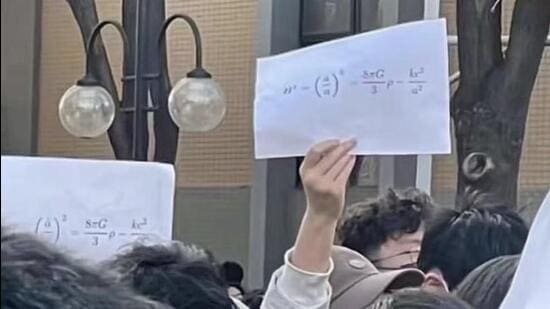# The Friedmann equations, and how they are related to protests in China

Updated on Nov 28, 2022 06:46 PM IST

## In images widely being circulated on social media, students of Beijing’s Tsinghua University can be seen holding sheets on which is written one of the Friedmann equations.Tsinghua University is a research university in northwest Beijing and is Chinese President Xi Jinping’s alma mater. (Twitter/Tkachuk_science)

NEW DELHI: Among all the protests that have erupted across China following the strict quarantine measures enforced by the government for Covid-19, one form that has stood out is the display of a physics equation.

In images widely being circulated on social media, students of Beijing’s Tsinghua University can be seen holding sheets on which is written one of the Friedmann equations.

What these equations have to do with the subject of the protests is open to speculation. Many on social media have suggested that it is a play on the words “free man”. Another view is that it symbolises a free and “open” China, because the Friedmann equations describe an “open” (expanding) universe.

What are the Friedmann equations

Named after the Russian physicist Alexander Friedmann, these are a set of equations that describe the rate at which the universe expands. In fact, Friedmann was the first physicist to predict that the universe is expanding.

The equation displayed in the protests in China is one form of the first Friedmann equation.

Simply put, the first Friedmann equation tells us the rate at which the universe is expanding. This, in turn, allows scientists to predict what the universe will look like at any point in the future, or work out what it looked like at any point in the past.

How the equations came to be

Before going into what the variables in the equation mean, it makes sense to understand the context in which these equations came.

Friedmann proposed this equation in 1922, having derived it from Albert Einstein’s 1915 Theory of General Relativity, but by reimagining the universe from the way Einstein saw it.

Also Read: China sees unprecedented protests against Covid curbs as cases rise

The General Theory describes the concept of “spacetime” and relates it with what we know as gravity. Einstein proposed that objects in the universe change the curvature of spacetime, and described a relationship among variables including mass, spacetime, energy and gravity. But if the theory had to work in a static universe, it needed a “fix”, which Einstein introduced in the form of a physical quantity he called the “cosmological constant”.

This is where Friedmann differed. While he used the General Theory itself, he proposed that the universe is homogeneous, isotropic (no fixed centre) and not static; it expands or contracts with time. From these assumptions emerged his equations.

The H in the equation is the expansion rate, known as the Hubble constant. The other variables describe quantities such as the gravitational constant, density and the speed of light.

Why it matters

While General Relativity was successful on many counts, including a reconfirmation of Newton’s gravity and a prediction of the bending of starlight, its “only problem”, according to the American astrophysicist and author Ethan Siegel, was the cosmological constant that Einstein introduced as an “ad hoc” fix. Otherwise, the assumption of static spacetimes would have caused the universe to collapse in on itself, Siegel explained in an article in Forbes magazine in 2018.

On the other hand, Seigel wrote, the Friedmann equations “tell you that the universe isn’t static, but rather that it either expands or contracts depending on what the expansion rate and the contents of your universe are. Best of all, they tell you how the universe evolves with time, arbitrarily far into the future or past”.

Incidentally, Einstein initially dismissed Friedmann’s equations as nothing more than a mathematical “curiosity”, according to a paper on the website of the University of North Carolina, Wilmington.

Get Latest World Newsalong with Latest Newsfrom Indiaat Hindustan Times.